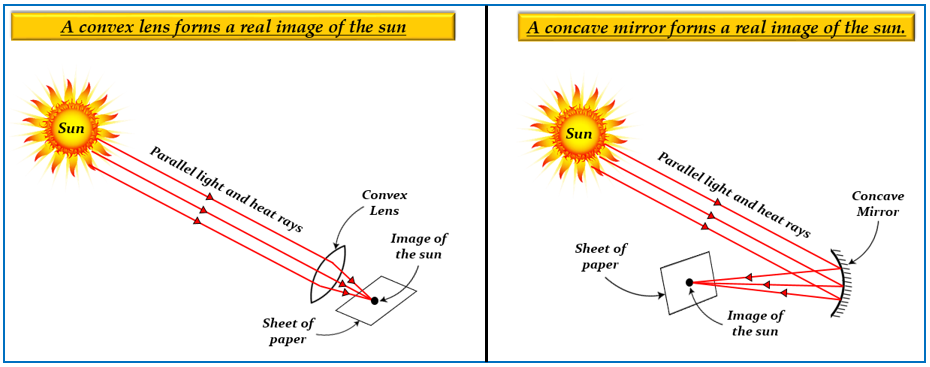# When sunlight is concentrated on a piece of paper by a spherical mirror or lens, then a hole can be burnt in it. For doing this, the paper must be placed at he focus of:(a) either a convex mirror or convex lens (b) either a concave mirror or concave lens(c) either a concave mirror or convex lens (d) either a convex mirror or concave lens

(c) either a concave mirror or a convex lens

Explanation

Both a concave mirror and a convex lens converge parallel beam of light coming from a distant object onto the focus $(F)$. In other words, a concave mirror and a convex lens form a real, inverted, and highly diminished image of an object at focus, when the object is at infinity.

Thus, light rays coming from the distant object i.e, the sun, converges at the focus $(f)$ of the concave mirror and a convex lens and forms a real, inverted, and diminished image of the sun. Since, these light rays have heat energy, which is when focused on a point of paper to create a sharp image of the sun, causing heat to increase at that point. Thus, the paper gets burnt on that point and leaves a hole.Updated on: 10-Oct-2022

116 Views